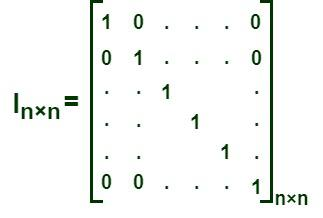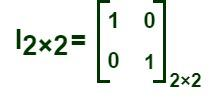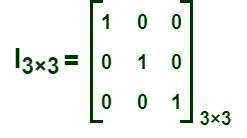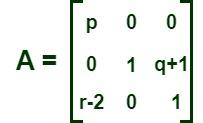Open in App
Not now

# Identity Matrix

• Last Updated : 02 Feb, 2023

A unit matrix, or identity matrix, is a square matrix whose all elements are zeros except the main diagonal elements which are ones. A matrix is defined as a rectangular array of numbers that are arranged in rows and columns. The size of a matrix can be determined by the number of rows and columns in it. A matrix is said to be an “m by n” matrix when it has “m” rows and “n” columns and is written as an “m × n” matrix. For example, the matrix given below is a “3 × 4” matrix, i.e., a matrix that has three rows and four columns. We have different types of matrices, such as rectangular matrices, square matrices, triangular matrices, symmetric matrices, etc.

## Identity Matrix Definition (Unit Matrix)

A unit matrix, or identity matrix, is a square matrix whose principal diagonal elements are ones, and the rest of the elements of the matrix are zeros. An identity matrix is always a square matrix and is expressed as “I.” For example, “In” is the identity matrix of order n, i.e., it has “n” rows and columns. It is a scalar matrix. When the product of any two square matrices is an identity matrix, then the matrices are said to be inverses of each other. The rank of an identity matrix of order “n × n” is n, as it has “n” linearly independent rows (or columns). The matrix given below represents an identity matrix of order “n by n.”Unit matrix of order n × n

### Examples of an Identity Matrix

A square matrix P = [aij] is said to be an identity matrix when aij = 1 for i = j and aij = 0 for i ≠ j.

⇒ The matrix given below is an identity matrix of order “2 × 2.”⇒ The matrix given below is an identity matrix of order “3 × 3.”## Properties of an Identity Matrix

The properties mentioned below are some important properties of an Identity Matrix:

• An identity matrix is always a square matrix, i.e., a matrix that has an equal number of rows and columns.
• The determinant of an identity matrix or unit matrix is always equal to 1, i.e., |I| = 1.

|I| = 1

• The inverse of an identity matrix or unit matrix is the identity matrix itself.

I-1 = I

• If an identity matrix of order “n” is multiplied by itself n times, then the resultant matrix will also be a unit matrix of order n.

In = I

• If any matrix A is multiplied by an identity matrix, then the resultant matrix is A.

AI = IA = A

• The product of two inverse matrices is an identity matrix.

AA-1 = A-1A = I

## Solved Examples on Identity Matrix

Example 1: Determine whether the given matrices are identity matrices or not.

a) A =b) B=Solution:

a) In matrix A, all the principal diagonal elements are ones, and the rest of the elements are also equal to 1. We know that a unit matrix, or identity matrix, is a square matrix whose all elements are zeros except the main diagonal elements, which are ones. Hence, matrix A is not an identity matrix.

b) In matrix B, all the principal diagonal elements are ones, and the rest of the elements are zeros. So, from the definition of an identity matrix, the given matrix B is an identity matrix.

Example 2: Give an example of an identity matrix that has four rows and four columns.

Solution:

The order of an identity matrix that has four rows and four columns is “4 × 4.” The matrix given below represents an identity matrix of order “4 × 4,” where all the principal diagonal elements are ones, and the rest of the elements are zeros.

I4×4Example 3: Find the value of (p − q + r) if the matrix given below is an identity matrix.Solution:

If the given matrix is an identity matrix, then all its principal diagonal elements are ones, and the rest of the elements are zeros.

So, p = 1

q + 1 = 0 ⇒ q = -1

r – 2 = 0 ⇒ r = 2

Now, p − q + r = 1 −(−1) + 2

= 1 + 1 + 2 = 4

Hence, the value of (p − q + r) is 4 if matrix A is an identity matrix.

Example 4: Prove that the inverse of the identity matrix is the identity matrix itself.

Solution:

Let’s consider an identity matrix of order “2 × 2” to prove that the inverse of the identity matrix is the identity matrix itself.

I2 × 2We know that the inverse of a matrix A= Adj A/ (ad – bc)

where Adj A =I-1 = 1/ (1 – 0)I-1= I

Hence proved.

Example 5: For a given matrix A, prove that AI = A.

Solution:

Let’s consider a square matrix A =to prove that AI = A.

AI === A

Hence, proved.

## FAQs on Identity Matrix

### Question 1: What is an identity matrix?

A unit matrix, or identity matrix, is a square matrix whose all elements are zeros except the main diagonal elements which are ones. A square matrix P = [aij] is said to be an identity matrix when aij = 1 for i = j and aij = 0 for i ≠ j.

### Question 2: Is an identity matrix a scalar matrix?

A scalar matrix is a square matrix whose principal diagonal elements are equal, and the rest of the elements of the matrix are zeros. We know that an identity matrix is a square matrix whose principal diagonal elements are ones, and the rest of the elements of the matrix are zeros. So, an identity matrix or a unit matrix is a scalar matrix.